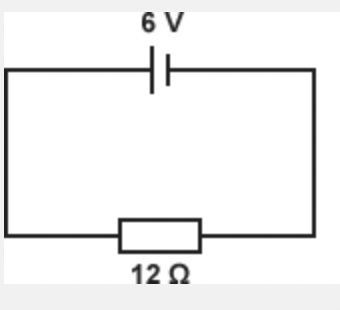# MCQ quiz on the Series and Parallel combination

This is an MCQ-based quiz on the topic of MCQ quiz on the Series and Parallel combination.

This set of MCQs helps you brush up on the important physics topics and prepare you to dive into skill practice and expand your knowledge.

Start Quiz

What is the current in this circuit?6A

2A

0.5A

0

An electronic device has a resistance of 20 ohms and a current of 15 A. What is the voltage across the device?

300V

30V

12V

3V

3−V potential difference is applied across a 6 resistor. What is the current that flows into the resistor?

0A

1A

0.5A

2A

A current of 0.2A passes through a 1.4kΩ resistor. What is the voltage across it?

200V

280V

300V

320V

A current of 0.2A passes through a 1.4kΩ resistor. What is the voltage across it?

200V

280V

300V

320V

In the circuit shown below, how much current does the ammeter show?1.25

2

13

0

In a circuit, the potential drop across the 10 kΩ -resistor is 100 V. What is the current through the resistor in A?

0.1

0.01

1

10

In the following circuits, find the unknowns.1200

120

12

1.0

An alarm clock draws 0.5 A current when connected to a 120 V circuit. Find its resistance.

240

250

200

260

A sub-woofer needs a household voltage of 110 V to push a current of 5.5 A through its coil. What is the resistance of the sub-woofer?

2

20

22

24

Quiz/Test Summary
Title: MCQ quiz on the Series and Parallel combination
Questions: 10
Contributed by: